Next: Gaugino Condensation Up: SUPERSTRING PHENOMENOLOGY AND THE Previous: Loop Corrections

# Model Independent Results

Let us in this section recapitulate what we can say about string models which is independent of the model. This is the closest we can get to string predictions and help us in approaching general questions, differentiating the generic issues from those of a particular model. Since the full nonperturbative formulation of string theory is not yet available, we have to content ourselves mostly with predictions of string perturbation theory, assuming that the corresponding string model is given buy a CFT.

(i) First, as it was mentioned in the introduction, 4D string models predict the existence of gravity and gauge interactions. This is a point that cannot be overemphasized since it is the first theory that makes those fundamental predictions for interactions we experience in the every day life. The dimension of spacetime is dynamical and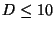raising the hope that eventually we could explain if a 4D spacetime is in some way special, although at present, the signature of spacetime is fixed in string theory as it is in QFT. Also the rank of the gauge group is bounded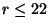(ii)There are other fields which survive at low energies: charged matter fields, candidates to be basic blocks of matter but also the dilaton field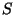and other moduli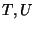. We have to mention that, although as yet there is no 4D model without moduli fields, there is not a general theorem implying their existence. In that sense the dilaton is the most generic modulus field, with a flat potential in perturbation theory.

(iii)There is only one arbitrary parameter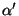fixed to be close to the Planck scale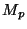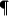. All other parameters of the effective action are determined by expectation values of fields such as the dilaton and the moduli. In particular the gauge coupling is given at tree level by the vev of.

(iv) The existence of spacetime supersymmetry is needed for consistency, although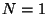is selected for phenomenological reasons. There is a general requirement for a CFT to lead tospacetime supersymmetry: It has to have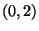supersymmetry in the wordlsheet (2D) (plus a quantization condition on the charges of the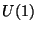group mixing the two supersymmetries) . Furthermore, it is not possible to break supersymmetry perturbatively nor in a continuous matter by the smooth variation of a parameter [57,58].(v) There are no global internal symmetries in 4D string models , besides the already mentioned Peccei-Quinn symmetry of thefield and some accidental global symmetries (like baryon and lepton numbers in the standard model). This is a very strong result derived by showing that if there is an internal symmetry, the properties of CFT's imply that there should be a vector field in the spectrum with the properties of the gauge field of that symmetry. This is consistent with similar claims about the nonexistence of global symmetries in gravity, due for instance to wormhole effects. This puts very strong constraints to string models compared with standard field theory models.

(vi)There are generically some discrete symmetries in string models. Some infinite dimensional such as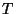-duality and others finite dimensional as those inherited from the point group of orbifold constructions, which are seen as discrete gauge symmetries. These can in principle be useful for model building, hierarchy of masses etc. There are however some couplings that vanish in string theory and cannot be explained in terms of symmetries of the effective 4D theory, these are called string miracles' since from the 4D point of view they seem to break the criterium for naturalness . As we saw in the previous section,-duality symmetries restrict very much the form of the effective action and quantities such as Yukawa couplings have to be modular forms of a given duality group. These symmetries are valid to all orders in string perturbation theory and are thought to be also preserved by nonperturbative effects. Matter fields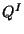are assigned special quantum numbers, the modular weights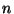, according to their transformation properties under the duality group. For a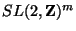group for instance we have: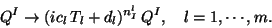(33)

Since fermions transform nontrivially under these symmetries, there may be duality anomalies' which have to be cancelled for consistency. This imposes strong constraints on the possible spectrum of the corresponding string model (which has to satisfy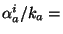constant). For instance, using this, it can be shown that it is impossible to obtain the minimal supersymmetric standard model spectrum from any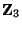or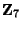orbifold models . Anomaly cancellation also implies that the dilatonhas to transform nontrivially under-duality, for factorized orbifolds: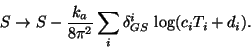(34)

(vii) There is unification without the need of a GUT. If the gauge group is a direct product of several groups we have for the heterotic string :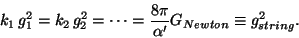(35)

Where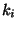are special stringy constants known as the Kac-Moody levels of the corresponding gauge groups (for the standard model groups it is usually assumed that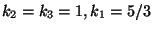). We can see there is a difference with standard GUTs in field theory for which we compute the unification scale by finding the point where the different string couplings meet. In heterotic string theory, the unification scale is given in terms of the string coupling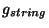and the Planck scale.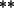More precisely: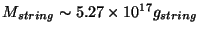Gev. For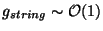this shows a discrepancy with the observed' value of the unification scale given by the experiments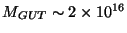GeV. Also the Weinberg angle gives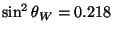differing from the experimental value of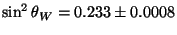. Therefore the string predictions' are very close to the experimental value, which is encouraging, but differ by several standard deviations from it. This is the string unification problem. The situation looks much better for simple GUT's which have good agreement with experiment. Several ideas have been proposed to cure this problem, including large values of threshold corrections, intermediate scales, extra particles, changing the values of Kac-Moody levels etc , with no compelling solution yet (for recent discussions of this issue see for example  and the review of K. Dienes in ref. .). Recently, a strong coupling solution of this problem was proposed which leads to a lower bound on Newton's constant, closed to the observed one .

(viii) Most of the models have Kac-Moody level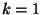and therefore cannot have adjoint representation particles. In order to have GUTs from strings, higher level models are then needed . These can be constructed from level-one models, for instance the breaking of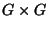to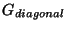leads to a level-two model. Some three and four generation string- GUTs have been explicitly constructed. General CFT relations show that in order to implement the missing partner' and see-saw mechanisms in string GUTs we would need Kac-Moody levels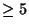. Also, if the breaking to the standard model by an adjoint is a flat direction then states transforming as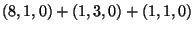under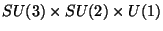remain in the light spectrum and could have low-energy implications.

(ix) There are usually fractionally charged particles in 4D string models . In fact it can be shown that we cannot have simultaneously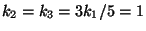in the standard model and only integer charged particles, because if that is the case the standard model gauge group would be enhanced to a full level-one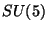. This problem' can be evaded in models where the fractionally charged particles are heavy string states, it has also been proposed that those particles could confine at intermediate energies and be unobservable .

(x)Nonrenormalization theorems of the previous sections are very strong and are model independent. We know the superpotential is exact at tree-level and the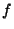function is exact at one-loop. The Kähler potential is however renormalized perturbatively. These results imply that the lifting of flat directions and the breaking of supersymmetry have to be achieved only at the string non-perturbative level (unless we do it by hand at tree-level  ).

(xi) There are anomalous'groups in most of the models, but there is also a counterterm in the action cancelling the anomaly and generating a Fayet-Iliopoulos kind of term :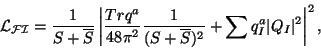(36)

where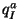are the anomalous charges of the scalar fields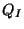. This term is responsable to break the would be anomalous group by fixing the value of a combination of the matter fields, breaking the would be anomalousand usually other gauge groups, but not supersymmetry. A combination of the fieldsand the dilatonstill remains massless and plays the role of the new dilaton field.

There are also further model independent results which refer to nonperturbative string effects and will be discussed next.

Supersymmetry Breaking 0.4cm

In this chapter we will approach the problem of supersymmetry breaking in string theories in two complementary ways. First we will discuss the favorite mechanism for low energy supersymmetry breaking, namely gaugino condensation, next we will try to extract general information about the effects of supersymmetry breaking, independent of the particular mechanism that breaks, we will then discuss the general form of soft breaking terms expected in string models, independent of the breaking mechanism. This is similar to the case of the standard model where the Higgs sector can be treated as a black box but we can study the low-energy theory below the symmetry breaking scale without relying on the particular mechanism that broke the symmetry. We will end up with general scenarios for the breaking of supersymmetry, discussing in particular the very generic problem, known as the cosmological moduli problem'.

We have seen that in the efforts to extract a relation between string theory and physics, there are two main problems, namely how the large vacuum degeneracy is lifted and how supersymmetry is broken at low energies. These problems, when present at string tree level, cannot be solved at any order in string perturbation theory. Therefore the only hope to solve them is nonperturbative physics. This has a good and a bad side. The good side is that nonperturbative effects represent the most natural way to generate large hierarchies due to their exponential suppression, this is precisely what is needed to obtain the Weinberg-Salam scale from the fundamental string or Planck scale. The bad side is that despite many efforts, we do not yet have a nonperturbative formulation of string theory. At the moment, the only concrete nonperturbative information we can extract is from the purely field theoretical nonperturbative effects inside string theory (although a great amount of progress has been made during the last year on nonperturbative string effects,  ). Probably the simplest and certainly the most studied of those effects is gaugino condensation in a hidden sector of the gauge group, since it has the potential of breaking supersymmetry as well as lifting some of the flat directions, as we will presently discuss.

SubsectionsNext: Gaugino Condensation Up: SUPERSTRING PHENOMENOLOGY AND THE Previous: Loop Corrections
root 2001-01-22